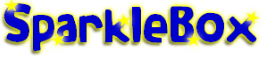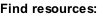﻿ Primary School Multiplication Teaching Resources and Activities - SparkleBox

HOME > Maths > Calculations > Multiplication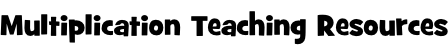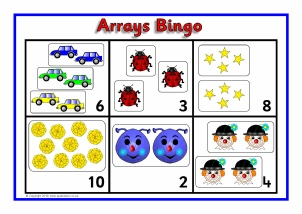Arrays (Multiplication) Bingo (SB3314)

Printable bingo boards with arrays of objects and multiplication number sentence cards to match.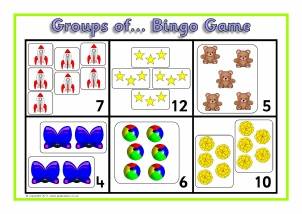Groups of... Arrays Bingo (SB6713)

Printable bingo boards with arrays of objects and ‘groups of...’ cards to match.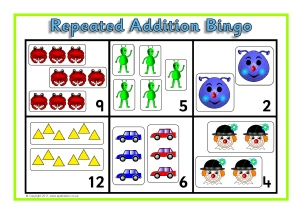Printable bingo boards with sets of objects and repeated addition number sentence cards to match.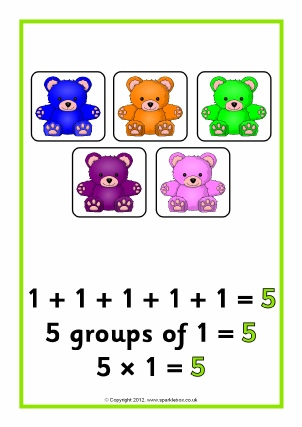Repeated Addition/Arrays Posters to 20 (SB8005)

Posters featuring pictorial representation of repeated addition/arrays for numbers to 20.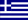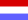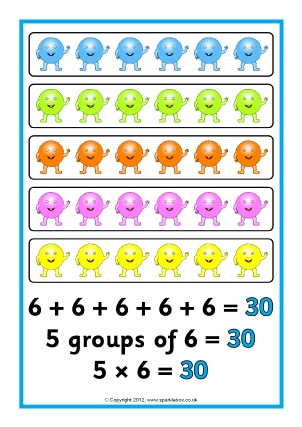(20-50) (SB8009)

Posters featuring pictorial representation of repeated addition/arrays for numbers in multiples of 5 from 20 to 50.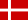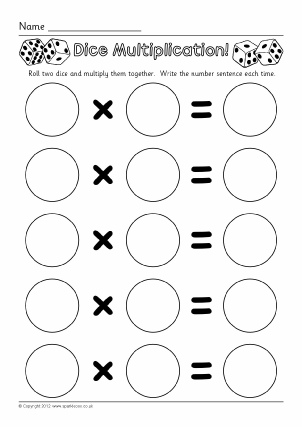Dice Multiplication Worksheets (SB7330)

Simple worksheets where pupils roll two dice, multiply the numbers together and write the number sentence.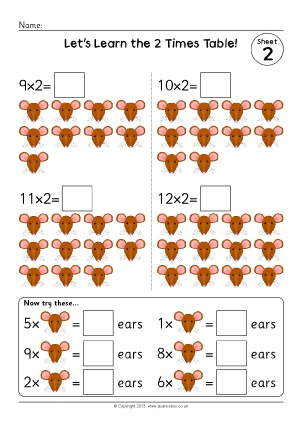2 Times Table Worksheets (SB9379)

A set of simple worksheets where children count the items and complete 2 times table number sentences.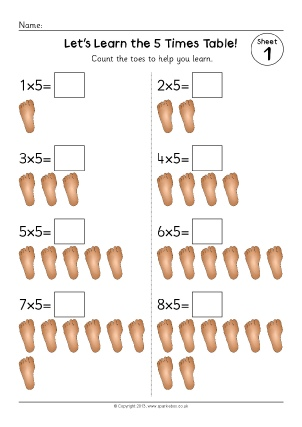5 Times Table Worksheets (SB9382)

A set of simple worksheets where children count the items and complete 3 times table number sentences.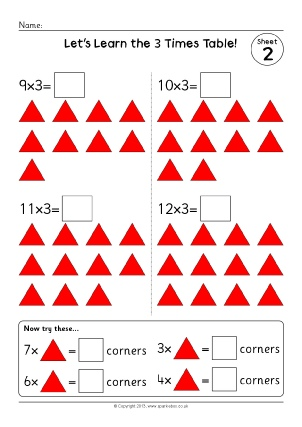3 Times Table Worksheets (SB9386)

A set of simple worksheets where children count the items and complete 3 times table number sentences.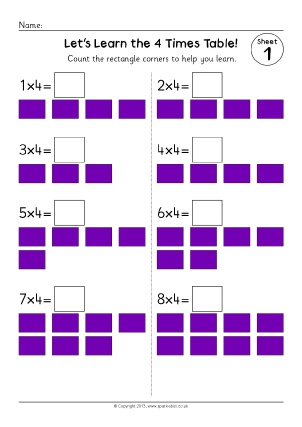4 Times Table Worksheets (SB9396)

A set of simple worksheets where children count the corners and complete 4 times table number sentences.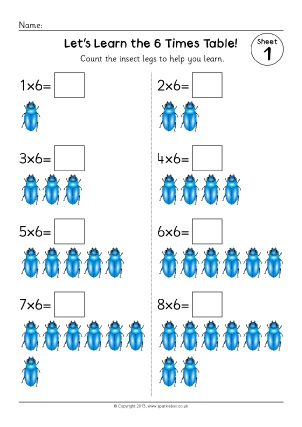6 Times Table Worksheets (SB9402)

A set of simple worksheets where children count the insect legs and complete 6 times table number sentences.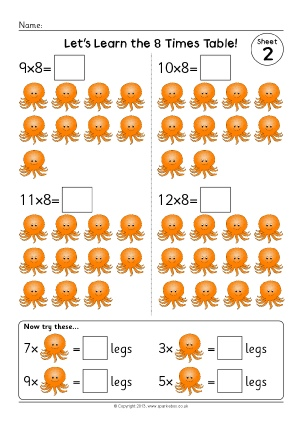8 Times Table Worksheets (SB9410)

A set of simple worksheets where children count the items and complete 8 times table number sentences.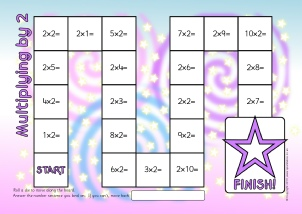Multiplying by 2 Board Game (SB9130)

A basic board game where children move around the board and answer the multiplication number sentences.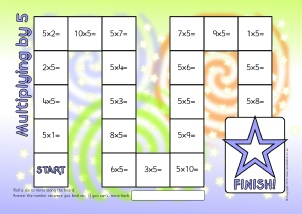Multiplying by 5 Board Game (SB9136)

A basic board game where children move around the board and answer the multiplication number sentences.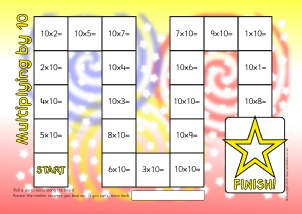Multiplying by 10 Board Game (SB9137)

A basic board game where children move around the board and answer the multiplication number sentences.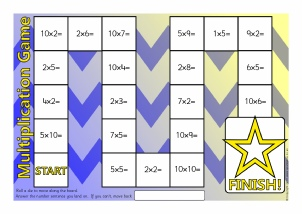Multiplying by 2, 5 and 10 Board Game (Ref: SB9147)

A basic board game where children move around the board and answer the multiplication number sentences.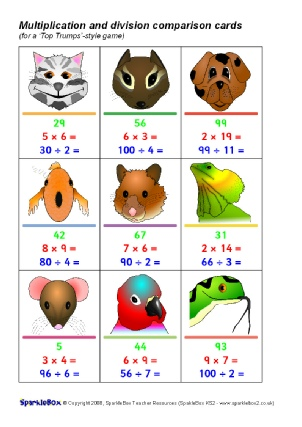Multiplication and Division ‘Top Trumps’ Style Game (SB6631)

A great set of 45 cards (9 per A4 page) for use in a ‘Top Trumps’-style game.  Children each have a hand of cards and take turns to compare either the number, multiplication or division so that the child with the highest result keeps the cards in each round.  Includes multiplication and division with results up to 100.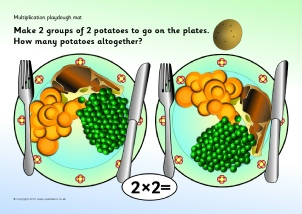Simple Multiplication Flash Cards - 2, 5 and 10 Times Tables (SB5629)

Simple flash cards featuring multiplication number sentences (without answers) for the 2, 5 and 10 times tables.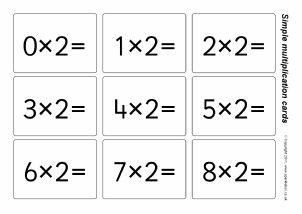Simple Multiplication Flash Cards - 2, 5 and 10 Times Tables (SB5629)

Simple flash cards featuring multiplication number sentences (without answers) for the 2, 5 and 10 times tables.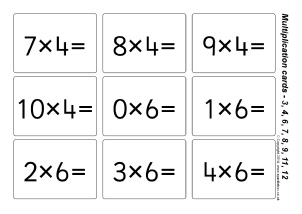Simple Multiplication Flash Cards - 3, 4, 6, 7, 8, 9, 11 and 12 Times Tables (SB10190)

Simple flash cards featuring multiplication number sentences (without answers) for the 3, 4, 6, 7, 8, 9, 11 and 12 times tables.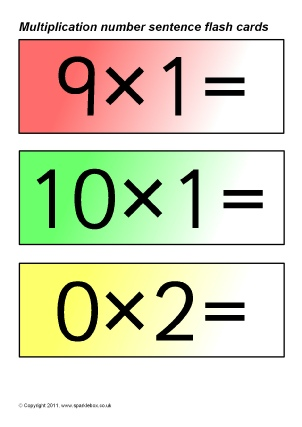Multiplication Number Sentence Flash Cards (SB5630)

Simple flash cards showing multiplication number sentences for the 1 to 10 times tables.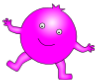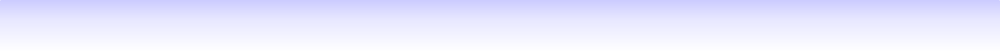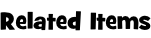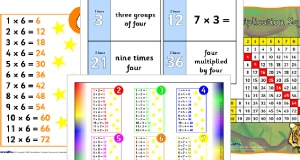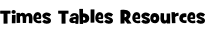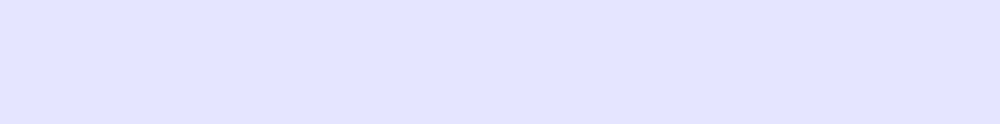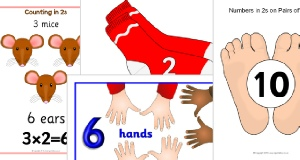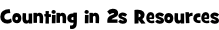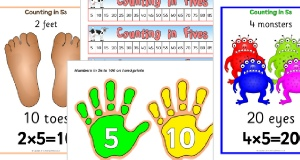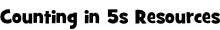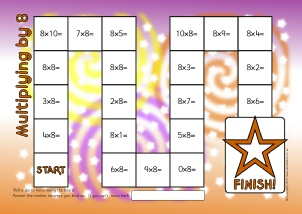Multiplying by 8 Board Game (SB11450)

A basic board game where children move around the board and answer the multiplication number sentences.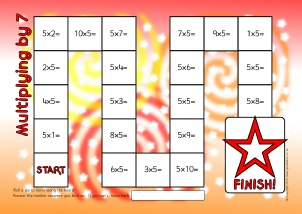Multiplying by 7 Board Game (SB11449)

A basic board game where children move around the board and answer the multiplication number sentences.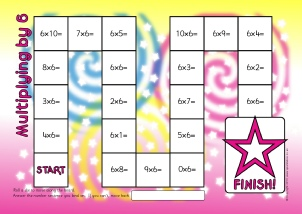Multiplying by 6 Board Game (SB11448)

A basic board game where children move around the board and answer the multiplication number sentences.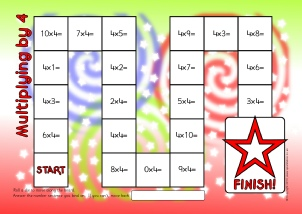Multiplying by 4 Board Game (SB11447)

A basic board game where children move around the board and answer the multiplication number sentences.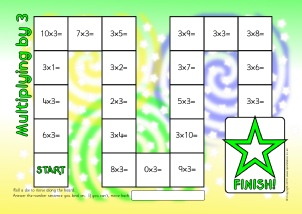Multiplying by 3 Board Game (SB11446)

A basic board game where children move around the board and answer the multiplication number sentences.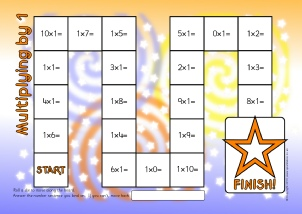Multiplying by 1 Board Game (SB11445)

A basic board game where children move around the board and answer the multiplication number sentences.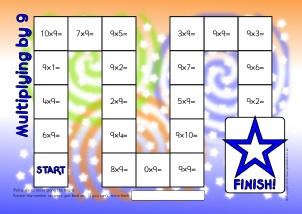Multiplying by 9 Board Game (SB11451)

A basic board game where children move around the board and answer the multiplication number sentences.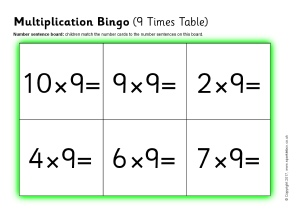Multiplication Bingo (9 Times Table) (SB12048)

A set of printable bingo boards and number cards with multiplication number sentences from the 9 times table.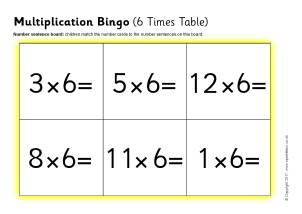Multiplication Bingo (6 Times Table) (SB12041)

A set of printable bingo boards and number cards with multiplication number sentences from the 6 times table.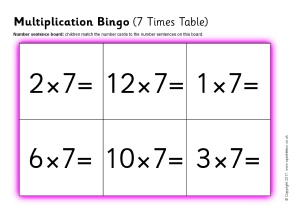Multiplication Bingo (7 Times Table) (SB12046)

A set of printable bingo boards and number cards with multiplication number sentences from the 7 times table.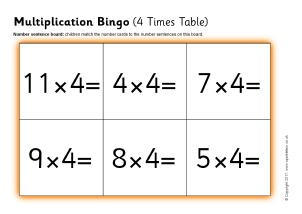Multiplication Bingo (4 Times Table) (SB12042)

A set of printable bingo boards and number cards with multiplication number sentences from the 4 times table.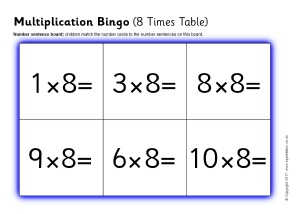Multiplication Bingo (8 Times Table) (SB12047)

A set of printable bingo boards and number cards with multiplication number sentences from the 8 times table.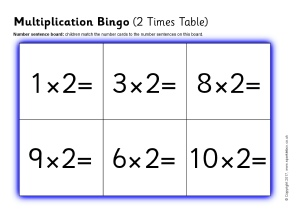Multiplication Bingo (2 Times Table) (SB12056)

A set of printable bingo boards and number cards with multiplication number sentences from the 2 times table.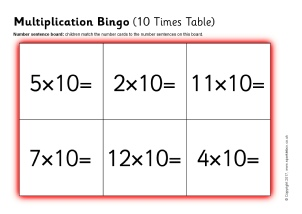Multiplication Bingo (10 Times Table) (SB12059)

A set of printable bingo boards and number cards with multiplication number sentences from the 10 times table.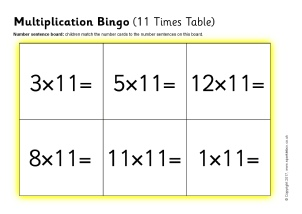Multiplication Bingo (11 Times Table) (SB12060)

A set of printable bingo boards and number cards with multiplication number sentences from the 11 times table.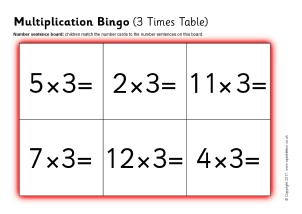Multiplication Bingo (3 Times Table) (SB12057)

A set of printable bingo boards and number cards with multiplication number sentences from the 3 times table.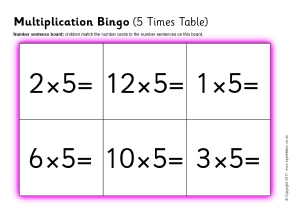Multiplication Bingo (5 Times Table) (SB12058)

A set of printable bingo boards and number cards with multiplication number sentences from the 5 times table.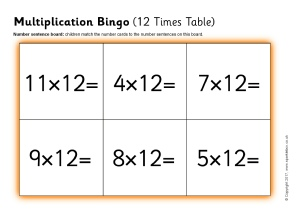Multiplication Bingo (12 Times Table) (SB12061)

A set of printable bingo boards and number cards with multiplication number sentences from the 12 times table.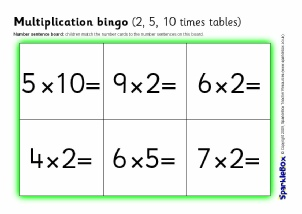Multiplication Bingo (2, 5, 10 Times Tables) (SB2341)

A set of printable bingo boards and number cards with multiplication number sentences.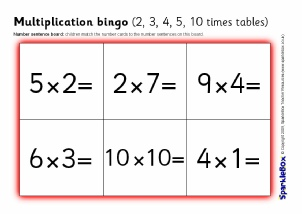Multiplication Bingo (2, 3, 4, 5 & 10 Times Tables) (SB6632)

A set of printable bingo boards and number cards with multiplication number sentences.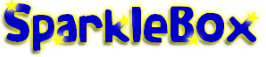New Literacy Maths Signs and Labels Class Management Special Needs Other Topics